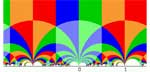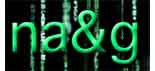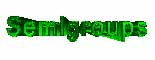# Tag: representationsCan
it be that one forgets an entire proof because the result doesn’t seem
important or relevant at the time? It seems the only logical explanation
for what happened last week. Raf Bocklandt asked me whether a
classification was known of all group algebras l G which are
noncommutative manifolds (that is, which are formally smooth a la Kontsevich-Rosenberg or, equivalently, quasi-free
a la Cuntz-Quillen). I said I didn’t know the answer and that it looked
like a difficult problem but at the same time it was entirely clear to
me how to attack this problem, even which book I needed to have a look
at to get started. And, indeed, after a visit to the library borrowing
Warren Dicks
lecture notes in mathematics 790 “Groups, trees and projective
modules” and browsing through it for a few minutes I had the rough
outline of the classification. As the proof is basicly a two-liner I
might as well sketch it here.
If l G is quasi-free it
must be hereditary so the augmentation ideal must be a projective
module. But Martin Dunwoody proved that this is equivalent to
G being a group acting on a (usually infinite) tree with finite
group vertex-stabilizers all of its orders being invertible in the
basefield l. Hence, by Bass-Serre theory G is the
fundamental group of a graph of finite groups (all orders being units in
l) and using this structural result it is then not difficult to
show that the group algebra l G does indeed have the lifting
property for morphisms modulo nilpotent ideals and hence is
quasi-free.
If l has characteristic zero (hence the
extra order conditions are void) one can invoke a result of Karrass
saying that quasi-freeness of l G is equivalent to G being
virtually free (that is, G has a free subgroup of finite
index). There are many interesting examples of virtually free groups.
One source are the discrete subgroups commensurable with SL(2,Z)
(among which all groups appearing in monstrous moonshine), another
source comes from the classification of rank two vectorbundles over
projective smooth curves over finite fields (see the later chapters of
Serre’s Trees). So
one can use non-commutative geometry to study the finite dimensional
representations of virtually free groups generalizing the approach with
Jan Adriaenssens in Non-commutative covers and the modular group (btw.
Jan claims that a revision of this paper will be available soon).
In order to avoid that I forget all of this once again, I’ve
written over the last couple of days a short note explaining what I know
of representations of virtually free groups (or more generally of
fundamental algebras of finite graphs of separable
l-algebras). I may (or may not) post this note on the arXiv in
the coming weeks. But, if you have a reason to be interested in this,
send me an email and I’ll send you a sneak preview.Tomorrow
in our masterclass. The idea of this course (and its companion
Projects in non-commutative algebra run by Fred Van Oystaeyen) is
that students should make a small (original if possible) work, that may
At this moment the students
have seen the following : definition and examples of quasi-free algebras
(aka formally smooth algebras, non-commutative curves), their
representation varieties, their connected component semigroup and the
Euler-form on it. Last week, Markus Reineke used all this in his mini-course
Rational points of varieties associated to quasi-free
algebras
. In it, Markus gave a method to compute (at least in
principle) the number of points of the non-commutative Hilbert
scheme
and the varieties of simple representations over a
finite field. Here, in principle means that Markus demands a lot of
knowledge in advance : the number of points of all connected components
of all representation schemes of the algebra as well as of its scalar
extensions over finite field extensions, together with the action of the
Galois group on them … Sadly, I do not know too many examples were all
this information is known (apart from path algebras of quivers).
Therefore, it seems like a good idea to run through Markus’
calculations in some specific examples were I think one can get all this
: free products of semi-simple algebras. The motivating examples
being the groupalgebra of the (projective) modular group
PSL(2,Z) = Z(2) * Z(3) and the free matrix-products $M(n,F_q) * M(m,F_q)$. I will explain how one begins to compute things in these
examples and will also explain how to get the One
quiver to rule them all
in these cases. It would be interesting to
compare the calculations we will find with those corresponding to the
path algebra of this one quiver.
As Markus set the good
example of writing out his notes and posting them, I will try to do the
same for my previous two sessions on quasi-free algebras over the next
couple of weeks.Again I
spend the whole morning preparing my talks for tomorrow in the master
class. Here is an outline of what I will cover :
– examples of
noncommutative points and curves. Grothendieck’s characterization of
commutative regular algebras by the lifting property and a proof that
this lifting property in the category alg of all l-algebras is
equivalent to being a noncommutative curve (using the construction of a
generic square-zero extension).
– definition of the affine
scheme rep(n,A) of all n-dimensional representations (as always,
l is still arbitrary) and a proof that these schemes are smooth
using the universal property of k(rep(n,A)) (via generic
matrices).
– whereas rep(n,A) is smooth it is in general
a disjoint union of its irreducible components and one can use the
sum-map to define a semigroup structure on these components when
l is algebraically closed. I’ll give some examples of this
semigroup and outline how the construction can be extended over
arbitrary basefields (via a cocommutative coalgebra).

definition of the Euler-form on rep A, all finite dimensional
representations. Outline of the main steps involved in showing that the
Euler-form defines a bilinear form on the connected component semigroup
when l is algebraically closed (using Jordan-Holder sequences and
upper-semicontinuity results).

After tomorrow’s
lectures I hope you are prepared for the mini-course by Markus Reineke on non-commutative Hilbert schemes
next week.Never thought that I would ever consider Galois descent of semigroup
coalgebras
but in preparing for my talks for the master-class it
came about naturally. Let A be a formally smooth algebra
(sometimes called a quasi-free algebra, I prefer the terminology
noncommutative curve) over an arbitrary base-field k. What, if
anything, can be said about the connected components of the affine
k-schemes rep(n,A) of n-dimensional representations
of A? If k is algebraically closed, then one can put a
commutative semigroup structure on the connected components induced by
the sum map

rep(n,A) x rep(m,A) -> rep(n + m,A)   :  (M,N)
-> M + N

as introduced and studied by Kent
Morrison
a long while ago. So what would be a natural substitute for
this if k is arbitrary? Well, define pi(n) to be the
maximal unramified sub k-algebra of k(rep(n,A)),
the coordinate ring of rep(n,A), then corresponding to the
sum-map above is a map

pi(n + m) -> pi(n) \\otimes
pi(m)

and these maps define on the graded
space

Pi(A) = pi(0) + pi(1) + pi(2) + ...

the
structure of a graded commutative k-coalgebra with
comultiplication

pi(n) -> sum(a + b=n) pi(a) \\otimes
pi(b)

The relevance of Pi(A) is that if we consider it
over the algebraic closure K of k we get the semigroup
coalgebra

K G  with  g -> sum(h.h\' = g) h \\otimes
h\'

where G is Morrison\’s connected component
semigroup. That is, Pi(A) is a k-form of this semigroup
coalgebra. Perhaps it is a good project for one of the students to work
this out in detail (and correct possible mistakes I made) and give some
concrete examples for formally smooth algebras A. If you know of
a reference on this, please let me know.Yesterday I made reservations for lecture rooms to run the
master class on non-commutative geometry sponsored by the ESF-NOG project. We have a lecture room on
monday- and wednesday afternoon and friday the whole day which should be
enough. I will run two courses in the program : non-commutative
geometry
and projects in non-commutative geometry both 30
hours. I hope that Raf Bocklandt will do most of the work on the
Geometric invariant theory course so that my contribution to it
can be minimal. Here are the first ideas of topics I want to cover in my
courses. As always, all suggestions are wellcome (just add a
comment).

non-commutative geometry : As
I am running this course jointly with Markus Reineke and as Markus will give a
mini-course on his work on non-commutative Hilbert schemes, I will explain
the theory of formally smooth algebras. I will cover most of the
paper by Joachim Cuntz and Daniel Quillen “Algebra extensions and
nonsingularity”, Journal of AMS, v.8, no. 2, 1995, 251?289. Further,
I’ll do the first section of the paper by Alexander Rosenberg and Maxim Kontsevich,
Noncommutative smooth spaces“. Then, I will
explain some of my own work including the “One
quiver to rule them all
” paper and my recent attempts to classify
all formally smooth algebras up to non-commutative birational
equivalence. When dealing with the last topic I will explain some of Aidan Schofield‘s paper
Birational classification of moduli spaces of representations of quivers“.

projects in
non-commutative geometry
: This is one of the two courses (the other
being “projects in non-commutative algebra” run by Fred Van Oystaeyen)
for which the students have to write a paper so I will take as the topic
of my talks the application of non-commutative geometry (in particular
the theory of orders in central simple algebras) to the resolution of
commutative singularities and ask the students to carry out the detailed
analysis for one of the following important classes of examples :
quantum groups at roots of unity, deformed preprojective algebras or
symplectic reflexion algebras. I will explain in much more detail three talks I gave on the subject last fall in
Luminy. But I will begin with more background material on central simple
algebras and orders.